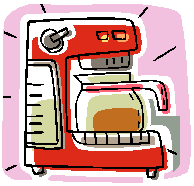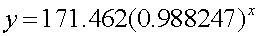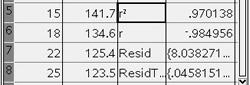Exponential Regression Model Example
(when doing an exponential regression, the y-values must be greater than 0)

Data:  The data at the right shows the cooling temperatures of a freshly brewed cup of coffee after it is poured from the brewing pot into a serving cup.  The brewing pot temperature is approximately 180º F.Time (mins)
Temp ( º F)
0
179.5
5
168.7
8
158.1
11
149.2
15
141.7
18
134.6
22
125.4
25
123.5
30
116.3
34
113.2
38
109.1
42
105.7
45
102.2
50
100.5
 Task: a.) Determine an exponential regression model equation to represent this data. b.) Graph the new equation. c.) Decide whether the new equation is a "good fit" to represent this data. d.) Based upon the new equation, what was the initial temperature of the coffee? e.) Interpolate data:  When is the coffee at a temperature of 106 degrees? f.) Extrapolate data:  What is the predicted temperature of the coffee after 1 hour? g.) In 1992, a woman sued McDonald's for serving coffee at a temperature of 180º  that caused her to be severely burned when the coffee spilled.  An expert witness at the trial testified that liquids at 180º will cause a full thickness burn to human   skin in two to seven seconds.  It was stated that had the coffee been served  at 155º, the liquid would have cooled and avoided the serious burns.  The  woman was awarded over 2.7 million dollars.  As a result of this famous case, many      restaurants now serve coffee at a temperature around 155º.   How long should restaurants wait (after pouring the coffee from the pot) before serving coffee, to ensure that the coffee is not hotter than 155º ? h.) If the temperature in the room is 76° F, what will happen to the temperature of the coffee, after being poured from the pot, over an extended period of time?

Step 1.  Enter the data into the lists.  Be sure to NAME the lists. For basic entry of data, see Lists & Spreadsheets.

Resize the columns to see the names.
MENU - #1 Actions - #2 Resize - #1Resize Column Widths
Use arrows to widen columns, hit ENTER when done, ESC.Step 2.  Create a scatter plot of the data.
Press HOME #5 Data & Statistics to create a generic plot of the data.

Move the cursor so that it hovers over "Click to add variable" at both the bottom and the left side of the screen.

Choose time for the x-variable and temp for the y-variable.Step 3.  Plot an Exponential Regression Model.
Choose MENU - #3 Actions - #5 Regression - Show ExponentialStep 4.  The graph of the Exponential Regression model appears.

Regression Equation:(answer to part a and part b)Step 5. Get statistical information.

Press MENU - #4 Statistics - #1 Stat Calculations - #A Exponential RegressionStep 6.
Choose X list: time
Choose Y list: temp
Save RegEqn to: f1Step 7. The statistical information will appear in Columns C and D. If you arrow down you will see the r value and the Residuals.Step 8. Is this model a "good fit"?
The correlation coefficient, r, is -.984956 which places the correlation into the "strong" category.  (0.8 or greater is a "strong" correlation)

The coefficient of determination, r 2, is .970138 which means that 97% of the total variation in y can be explained by the relationship between x and y
Yes, it is a very "good fit".Step 9. Based upon the new equation, what was the initial temperature of the coffee?

The exponential regression equation iswhere x stands for time.  The initial temperature would occur when the time equals zero.  Substituting zero for x gives an initial temperature of 171.462º.

Step 10. Interpolate:  (within the data set)
When is the coffee at a temperature of 106 degrees?

We need a table of values so we can investigate other options.

MENU - #5 Function Table - #1 Switch to Function Table (or CTRL T) - ENTER

Choose f(x) f1 since we stored the regression equation in f1.Look through the table to get an idea of approximately where the 106 temperature may occur. It is around a time of 40.

To change the table settings to get a more accurate reading, choose MENU - #5 Function Table - #3 Edit Function Table Settings
Start the table at 40 and set delta table to .001. Find the value.

(answer to part e --  after 40.678 minutes)Step 11. Extrapolate data: (beyond the data set)
What is the predicted temperature of the coffee after 1 hour?

Press HOME - #1 Calculator. Since we stored the regression equation in f1, we can use this function to find values for making predictions.
(answer to part f -- 84.3522º F)Step 12.   How long should the restaurants wait (after pouring the coffee from the pot) before serving coffee, to ensure that the coffee is not hotter than 155º ?

Repeat procedure from Step 10. Go back to that page and reset the Function Table settings:

(answer to part g -- approx. 8.5  minutes)Step 13.  If the temperature in the room is 76° F, what will happen to the temperature of the coffee, after being poured from the pot, over an extended period of time?

The new exponential equation shows that the coffee will reach room temperature after 68.8 minutes.  The exponential graph is asymptotic to the x-axis, therefore the y-values approach zero.  This can be seen by observing successively larger values substituted into the new exponential equation (see Calculator screen screen substitutions at the right).

Even though the graph approaches zero asymptotically, the temperature of the coffee will stop cooling once it reaches room temperature of 76º, and will not continue following the curve of the graph.  (Also, remember that when extrapolating data, the further away from the data set you go, the less reliable your new data becomes.)